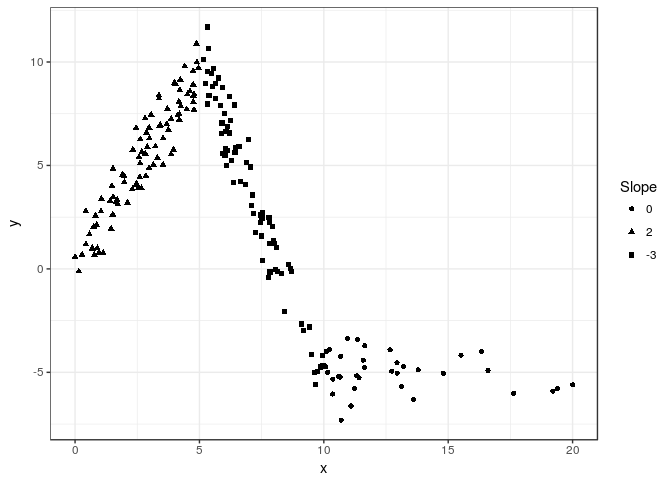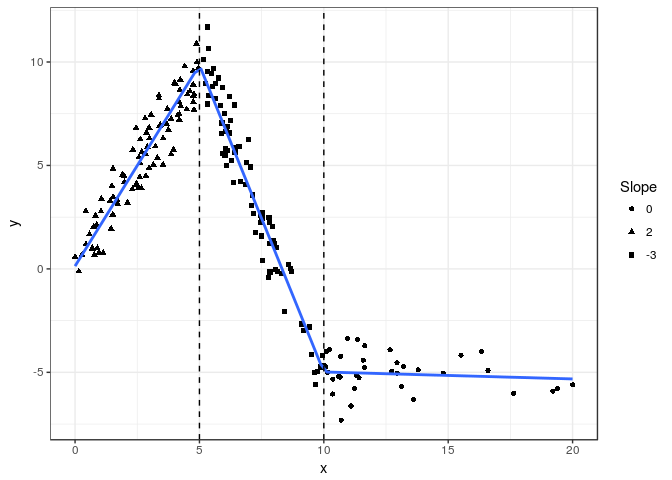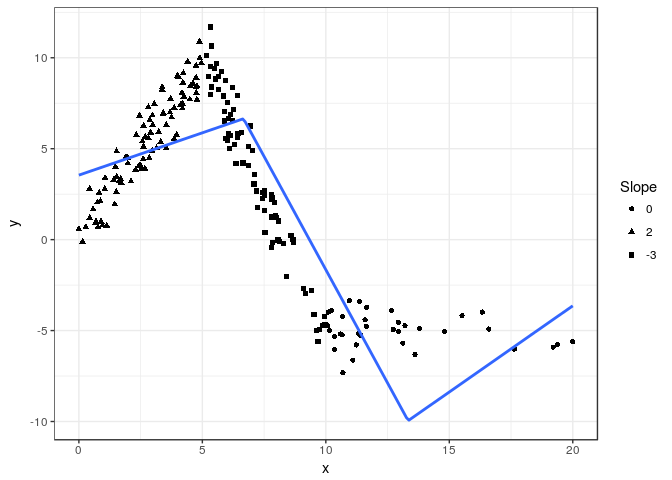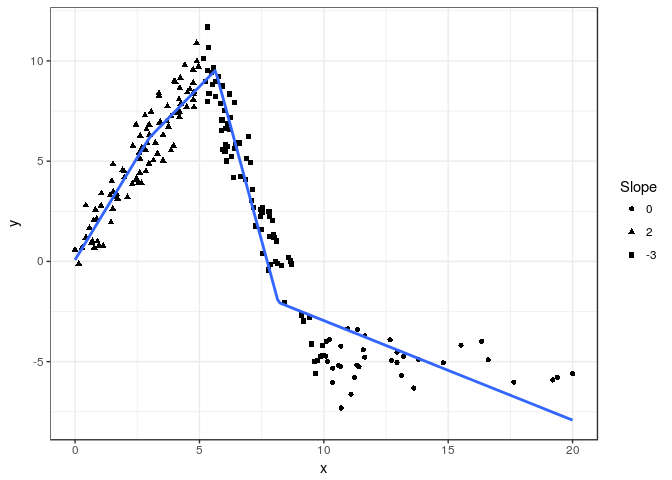# lspline: Linear Splines with Convenient Parametrisations

Linear splines with convenient parametrisations such that

• coefficients are slopes of consecutive segments
• coefficients capture slope change at consecutive knots

Knot locations can be specified

• manually (`lspline()`)
• at breaks dividing the range of `x` into `q` equal-frequency intervals (`qlspline()`)
• at breaks dividing the range of `x` into `n` equal-width intervals (`elspline()`)

# Examples

Examples of using `lspline()`, `qlspline()`, and `elspline()`. We will use the following artificial data with knots at `x=5` and `x=10`

``````set.seed(666)
n <- 200
d <- data.frame(
x = scales::rescale(rchisq(n, 6), c(0, 20))
)
d\$interval <- findInterval(d\$x, c(5, 10), rightmost.closed = TRUE) + 1
d\$slope <- c(2, -3, 0)[d\$interval]
d\$intercept <- c(0, 25, -5)[d\$interval]
d\$y <- with(d, intercept + slope * x + rnorm(n, 0, 1))``````

Plotting `y` against `x`:

``````library(ggplot2)
fig <- ggplot(d, aes(x=x, y=y)) +
geom_point(aes(shape=as.character(slope))) +
scale_shape_discrete(name="Slope") +
theme_bw()
fig``````The slopes of the consecutive segments are 2, -3, and 0.

## Setting knot locations manually

We can parametrize the spline with slopes of individual segments (default `marginal=FALSE`):

``````library(lspline)
m1 <- lm(y ~ lspline(x, c(5, 10)), data=d)
knitr::kable(broom::tidy(m1))``````
term estimate std.error statistic p.value
(Intercept) 0.1343204 0.2148116 0.6252941 0.5325054
lspline(x, c(5, 10))1 1.9435458 0.0597698 32.5171747 0.0000000
lspline(x, c(5, 10))2 -2.9666750 0.0503967 -58.8664832 0.0000000
lspline(x, c(5, 10))3 -0.0335289 0.0518601 -0.6465255 0.5186955

Or parametrize with coeficients measuring change in slope (with `marginal=TRUE`):

``````m2 <- lm(y ~ lspline(x, c(5,10), marginal=TRUE), data=d)
knitr::kable(broom::tidy(m2))``````
term estimate std.error statistic p.value
(Intercept) 0.1343204 0.2148116 0.6252941 0.5325054
lspline(x, c(5, 10), marginal = TRUE)1 1.9435458 0.0597698 32.5171747 0.0000000
lspline(x, c(5, 10), marginal = TRUE)2 -4.9102208 0.0975908 -50.3143597 0.0000000
lspline(x, c(5, 10), marginal = TRUE)3 2.9331462 0.0885445 33.1262479 0.0000000

The coefficients are

• `lspline(x, c(5, 10), marginal = TRUE)1` - the slope of the first segment
• `lspline(x, c(5, 10), marginal = TRUE)2` - the change in slope at knot x = 5; it is changing from 2 to -3, so by -5
• `lspline(x, c(5, 10), marginal = TRUE)3` - tha change in slope at knot x = 10; it is changing from -3 to 0, so by 3

The two parametrisations (obviously) give identical predicted values:

``````all.equal( fitted(m1), fitted(m2) )
##  TRUE``````

graphically

``````fig +
geom_smooth(method="lm", formula=formula(m1), se=FALSE) +
geom_vline(xintercept = c(5, 10), linetype=2)``````## Knots at `n` equal-length intervals

Function `elspline()` sets the knots at points dividing the range of `x` into `n` equal length intervals.

``````m3 <- lm(y ~ elspline(x, 3), data=d)
knitr::kable(broom::tidy(m3))``````
term estimate std.error statistic p.value
(Intercept) 3.5484817 0.4603827 7.707678 0.00e+00
elspline(x, 3)1 0.4652507 0.1010200 4.605529 7.40e-06
elspline(x, 3)2 -2.4908385 0.1167867 -21.328105 0.00e+00
elspline(x, 3)3 0.9475630 0.2328691 4.069080 6.84e-05

Graphically

``````fig +
geom_smooth(aes(group=1), method="lm", formula=formula(m3), se=FALSE, n=200)``````## Knots at `q`uantiles of `x`

Function `qlspline()` sets the knots at points dividing the range of `x` into `q` equal-frequency intervals.

``````m4 <- lm(y ~ qlspline(x, 4), data=d)
knitr::kable(broom::tidy(m4))``````
term estimate std.error statistic p.value
(Intercept) 0.0782285 0.3948061 0.198144 0.8431388
qlspline(x, 4)1 2.0398804 0.1802724 11.315548 0.0000000
qlspline(x, 4)2 1.2675186 0.1471270 8.615132 0.0000000
qlspline(x, 4)3 -4.5846478 0.1476810 -31.044273 0.0000000
qlspline(x, 4)4 -0.4965858 0.0572115 -8.679818 0.0000000

Graphically

``````fig +
geom_smooth(method="lm", formula=formula(m4), se=FALSE, n=200)``````# Installation

Stable version from CRAN or development version from GitHub with

``devtools::install_github("mbojan/lspline", build_vignettes=TRUE)``

# Acknowledgements

Inspired by Stata command `mkspline` and function `ares::lspline` from Junger & Ponce de Leon (2011). As such, the implementation follows Greene (2003), chapter 7.5.2.

• Greene, William H. (2003) Econometric analysis. Pearson Education
• Junger & Ponce de Leon (2011) `ares`: Environment air pollution epidemiology: a library for timeseries analysis. R package version 0.7.2 retrieved from CRAN archives.